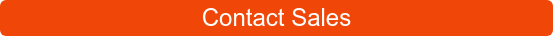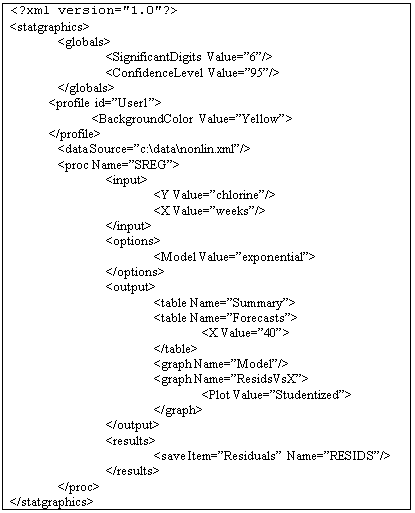# Statgraphics Web Services

STATGRAPHICS Web Services are designed to be called by user-written web applications to perform various statistical calculations and return tabular and graphical results. In a typical implementation, a web application creates an XML script which is sent to the web service together with the data to be analyzed. The web service processes the request and returns XML results, HTML tables, or graphics images for the web application to display.

STATGRAPHICS stratus software is an example of a web application that accesses the STATGRAPHICS Web Services.

Pricing for STATGRAPHICS Web Services software:# XML Scripts

User-written web applications communicate with the web services using the STATGRAPHICS XML Scripting Language. Using the scripting language, system globals can be set, data files can be accessed, and statistical procedures can be invoked. Scripts developed with the XML scripting language may be executed by:

1. STATGRAPHICS Centurion Version XVI or later.

2. The STATGRAPHICS Online Web Services.

A STATGRAPHICS XML script is a text file containing XML instructions similar to the following:The various sections of the script set global options and graphics parameters, describe the data source, and specify the statistical procedures to be performed.

# Contents

The following procedures and data analysis tools are currently available in the STATGRAPHICS Online Web Services:

Name Menu selection Description
ANOVA Multifactor ANOVA Builds a model involving several categorical factors.
BARCHART Barchart Displays a barchart for a column of counts.
BOXPLOT Box-and-Whisker Plot Creates a box-and-whisker plot for a single sample.
BUBBLECHART Bubble Chart Creates a chart with circlesov varying size.

CAPABILITY

CAPABILITYGROUPED

Capability Analysis for Individual and Grouped Data Performs a process capability analysis to compare data to a set of specifications.
CCHART Control Chart for Counts Creates a control chart for data from a Poisson distribution.
CONTINGENCY Contingency Tables Displays two-way table of counts.
CROSSTABULATE Crosstabulation Constructs two-way tables from two categorical data columns.
DATATABLE Print Datasheet Displays contents of current data table.
DECOMPOSITION Seasonal Decomposition Performs a seasonal adjustment of a time series and calculates seasonally adjusted values.
DISTFIT Distribution Fitting Fits distributions to uncensored data.
FORECAST User-Specified Forecast Models Fits and forecasts using specified time series models.
FTABLE Frequency Table Displays charts for a column of counts.
HISTOGRAM Frequency Histogram Creates a histogram for a single sample.
MATRIXPLOT Matrix Plot Creates a scatterplot matrix.
MBARCHART Multiple Barchart Displays a barchart for a twoway table of counts.
MBOXPLOT Multiple Box-and-Whisker Plot Creates a box-and-whisker plot for a multiple samples.
MEDIANRCHART Median and R Control Charts Creates control charts for the median and range.
MREG Multiple Regression Fits linear models relating Y to multiple X’s.
MULTSAM Multiple Sample Comparison Compares numeric data in two or more columns.
MULTVAR Multiple Variable Analysis Calculates statistics for two or more numeric variables.
MXYPLOT Multiple X-Y Plot Plots two or more Y columns versus a common X.
MXYZPLOT Multiple X-Y-Z Plot Plots two or more Z columns versus a common X and Y.
NORMALPLOT Normal Probability Plot Plot a normal probability plot for a single sample.
NPCHART Control Chart for Counts Creates a control chart for data from a binomial distribution.
ONEVAR One Variable Analysis Calculates statistics for a single column of numeric data.
ONEWAY Oneway ANOVA Determines the relationship between a numeric response variable and one categorical factor.
PAIRED Paired Sample Comparison Compares numeric data in two or more columns, when the data in each row are paired.
PARETO Pareto Analysis Creates a Pareto chart to illustrate the vital few causes of defects.
PCHART Control Chart for Proportions Creates a control chart for data from a binomial distribution.
PIECHART Piechart Displays a piechart for a column of counts.
PROBDIST Probability Distributions Calculates and displays probability distributions.
QUANTILEPLOT Quantile Plot Plots the empirical CDF for a column of numeric data.
SAMPDIST Sampling Distributions Calculates tail areas and critical values for common sampling distributions.
SIXSIGMA Six Sigma Indices Converts between various Six Sigma measures of quality.
SMOOTH Time Series Smoothing Smoothes time series data using various linear and nonlinear smoothers.
SREG Simple Regression Fits linear and nonlinear models relating Y to X.
TABULATE Tabulation Calculates statistics for a single column of categorical data.
TIMESERIES Descriptive Time Series Methods Plots time series data and calculates descriptive statistics.
TWOSAM Two Sample Comparison Compares numeric data in two or more columns.
UCHART Control Chart for Rates Creates a control chart for data from a Poisson distribution.
USMAP United States Dempgraphic Map Plots demographics data on a state-by-state basis.
WEIBULL Weibull Analysis Performs a Weibull analysis on reliability data.
XBARRCHART X-bar and R Control Charts Creates control charts for the mean and range.
XBARSCHART X-bar and S Control Charts Creates control charts for the mean and standard deviation.
XBARSSCHART X-bar and S-Squared Control Charts Creates control charts for the mean and variance.
XCHART Individual and Moving Range Control Chart Control control charts for individuals data and a moving range of 2.
XPLOT X Plot Scatterplot for a single numeric column.
XYPLOT X-Y Plot Scatterplot for two numeric columns.
XYZPLOT X-Y-Z Plot Scatterplot for three numeric columns.